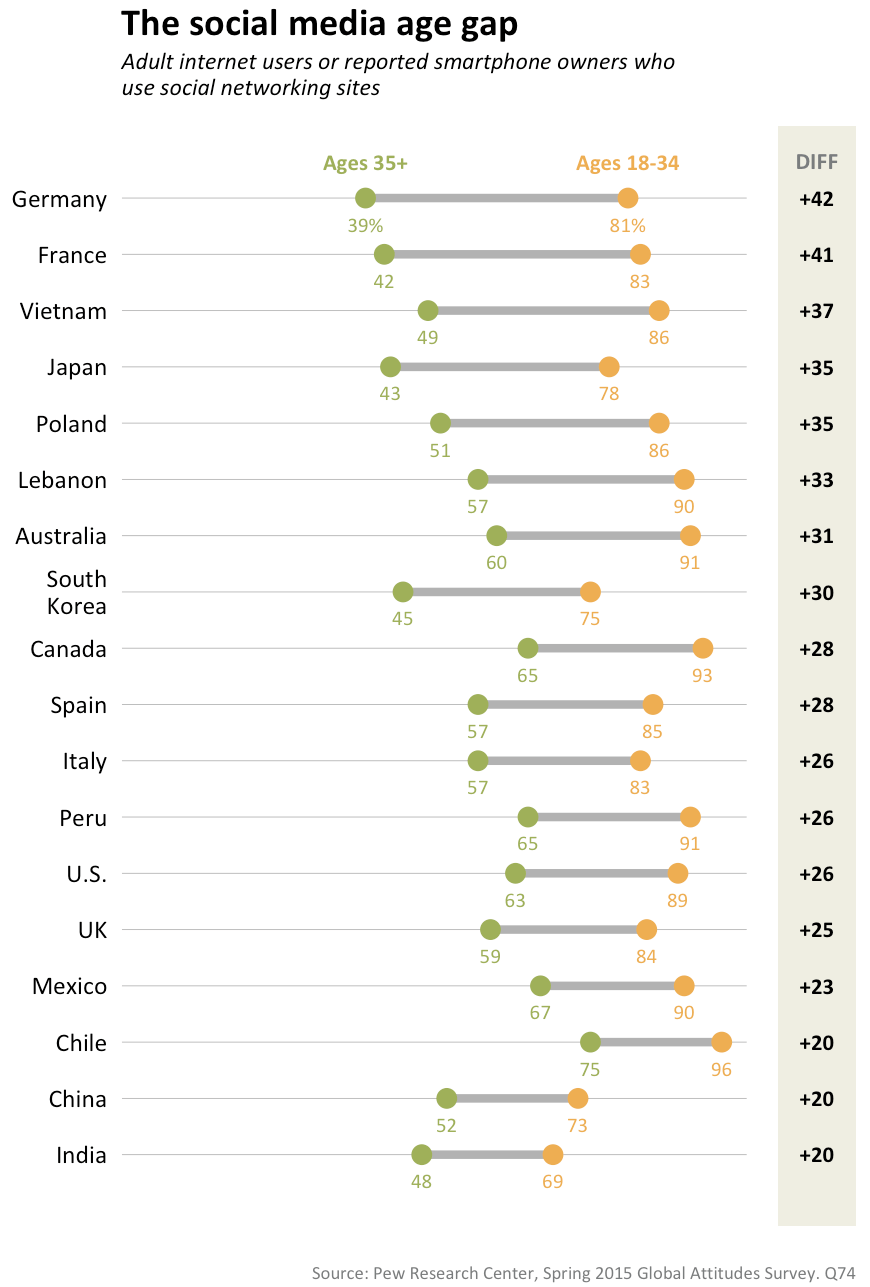Want to share your content on R-bloggers? click here if you have a blog, or here if you don't.

I follow the most excellent Pew Research folks on Twitter to stay in tune with what’s happening (statistically speaking) with the world. Today, they tweeted this excerpt from their 2015 Global Attitudes survey:

I thought it might be helpful to folks if I made a highly aesthetically tuned version of Pew’s chart (though I chose to go a bit more minimal in terms of styling than they did) with the new `geom_dumbbell()` in the development version of `ggalt`. The source (below) is annotated, but please drop a note in the comments if any of the code would benefit from more exposition.

I’ve also switched to using the Prism javascript library starting with this post after seeing how well it works in RStudio’s `flexdashboard` package. If the “light on black” is hard to read or distracting, drop a note here and I’ll switch the theme if enough folks are having issues.

```library(ggplot2) # devtools::install_github("hadley/ggplot2")
library(ggalt)   # devtools::install_github("hrbrmstr/ggalt")
library(dplyr)   # for data_frame() & arrange()

# I'm not crazy enough to input all the data; this will have to do for the example
df <- data_frame(country=c("Germany", "France", "Vietnam", "Japan", "Poland", "Lebanon",
"Australia", "SouthnKorea", "Canada", "Spain", "Italy", "Peru",
"U.S.", "UK", "Mexico", "Chile", "China", "India"),
ages_35=c(0.39, 0.42, 0.49, 0.43, 0.51, 0.57,
0.60, 0.45, 0.65, 0.57, 0.57, 0.65,
0.63, 0.59, 0.67, 0.75, 0.52, 0.48),
ages_18_to_34=c(0.81, 0.83, 0.86, 0.78, 0.86, 0.90,
0.91, 0.75, 0.93, 0.85, 0.83, 0.91,
0.89, 0.84, 0.90, 0.96, 0.73, 0.69),
diff=sprintf("+%d", as.integer((ages_18_to_34-ages_35)*100)))

# we want to keep the order in the plot, so we use a factor for country
df <- arrange(df, desc(diff))
df\$country <- factor(df\$country, levels=rev(df\$country))

# we only want the first line values with "%" symbols (to avoid chart junk)
# quick hack; there is a more efficient way to do this
percent_first <- function(x) {
x <- sprintf("%d%%", round(x*100))
x[2:length(x)] <- sub("%\$", "", x[2:length(x)])
x
}

gg <- ggplot()
# doing this vs y axis major grid line
gg <- gg + geom_segment(data=df, aes(y=country, yend=country, x=0, xend=1), color="#b2b2b2", size=0.15)
# dum…dum…dum!bell
gg <- gg + geom_dumbbell(data=df, aes(y=country, x=ages_35, xend=ages_18_to_34),
size=1.5, color="#b2b2b2", point.size.l=3, point.size.r=3,
point.colour.l="#9fb059", point.colour.r="#edae52")
# text below points
gg <- gg + geom_text(data=filter(df, country=="Germany"),
aes(x=ages_35, y=country, label="Ages 35+"),
color="#9fb059", size=3, vjust=-2, fontface="bold", family="Calibri")
gg <- gg + geom_text(data=filter(df, country=="Germany"),
aes(x=ages_18_to_34, y=country, label="Ages 18-34"),
color="#edae52", size=3, vjust=-2, fontface="bold", family="Calibri")
# text above points
gg <- gg + geom_text(data=df, aes(x=ages_35, y=country, label=percent_first(ages_35)),
color="#9fb059", size=2.75, vjust=2.5, family="Calibri")
gg <- gg + geom_text(data=df, color="#edae52", size=2.75, vjust=2.5, family="Calibri",
aes(x=ages_18_to_34, y=country, label=percent_first(ages_18_to_34)))
# difference column
gg <- gg + geom_rect(data=df, aes(xmin=1.05, xmax=1.175, ymin=-Inf, ymax=Inf), fill="#efefe3")
gg <- gg + geom_text(data=df, aes(label=diff, y=country, x=1.1125), fontface="bold", size=3, family="Calibri")
gg <- gg + geom_text(data=filter(df, country=="Germany"), aes(x=1.1125, y=country, label="DIFF"),
color="#7a7d7e", size=3.1, vjust=-2, fontface="bold", family="Calibri")
gg <- gg + scale_x_continuous(expand=c(0,0), limits=c(0, 1.175))
gg <- gg + scale_y_discrete(expand=c(0.075,0))
gg <- gg + labs(x=NULL, y=NULL, title="The social media age gap",
subtitle="Adult internet users or reported smartphone owners whonuse social networking sites",
caption="Source: Pew Research Center, Spring 2015 Global Attitudes Survey. Q74")
gg <- gg + theme_bw(base_family="Calibri")
gg <- gg + theme(panel.grid.major=element_blank())
gg <- gg + theme(panel.grid.minor=element_blank())
gg <- gg + theme(panel.border=element_blank())
gg <- gg + theme(axis.ticks=element_blank())
gg <- gg + theme(axis.text.x=element_blank())
gg <- gg + theme(plot.title=element_text(face="bold"))
gg <- gg + theme(plot.subtitle=element_text(face="italic", size=9, margin=margin(b=12)))
gg <- gg + theme(plot.caption=element_text(size=7, margin=margin(t=12), color="#7a7d7e"))
gg```